Technical Article

# Conversion of T Flip-Flops

September 14, 2016 by Sneha H.L.

## Here we convert the given T flip-flop into SR-, JK- and D-types, and we also verify the process of conversion.

Here we convert the given T flip-flop into SR-, JK- and D-types, and we also verify the process of conversion.

### Introduction

This article covers the steps involved in converting a given T flip-flop into SR-, JK-, and D-type flip-flops. We also present a verification technique for these conversions; the verification process allows us to ensure that the designed systems provide the desired functionality.

### Previous Articles in This Series

Please refer to the previous parts in this series, particularly the first two, for a detailed explanation of the process:

### Conversion of a T to an SR Flip-Flop

In order to convert a given T flip-flop into SR-type, we need to combine the information presented in the SR flip-flop's truth table and the information in the T flip-flop's excitation table into a common table. This can be referred to as a T-to-SR conversion table and is as shown in Figure 1.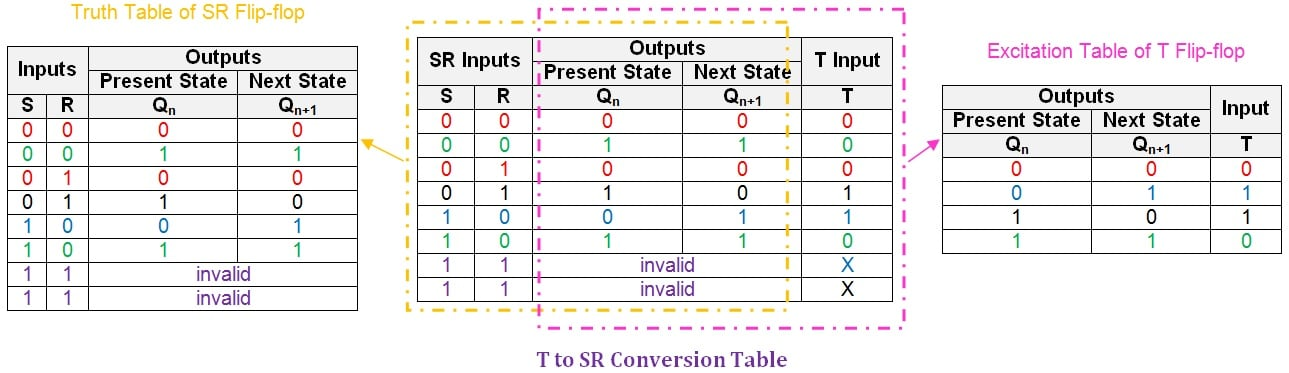##### Figure 1: T-to-SR conversion table. Click to enlarge.

Notice the don't care (X) entries in the last two rows of the conversion table's "T input" column. These indicate that when both inputs (S and R) are driven high, the output of the SR flip-flop is unpredictable (owing to the "race around condition").

Next, we should express the input of the given flip-flop in terms of the present-state, Qn, and the input(s) of the desired flip-flop. This can be done by using a suitable simplification technique, such as the K-map (discussed in detail in a separate article).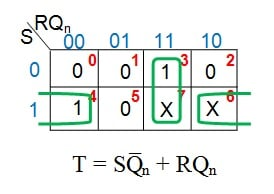##### Figure 2: K-map simplification for the T input in terms of S, R, and Qn

Figure 2 shows that the simplified logical expression for the T input in terms of S, R, and Qn is SQ̅n + RQn.

To make the given T flip-flop functionally equivalent to the desired SR flip-flop, we need to AND Q̅n with the user-defined input S and also AND Qn with the user-provided input R. The results of these AND operations are then ORed together.

Thus, we require two AND gates and one OR gate to convert the T flip-flop to an SR-type, as shown in Figure 3.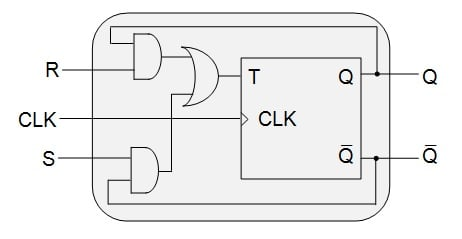### Verification

Having designed the system, we will now verify that the conversion process was successful. This can be accomplished by means of the truth table–based verification technique. The process involves comparison between the truth table of the desired (SR) flip-flop and the verification table for the T-to-SR conversion, as shown in Figure 4.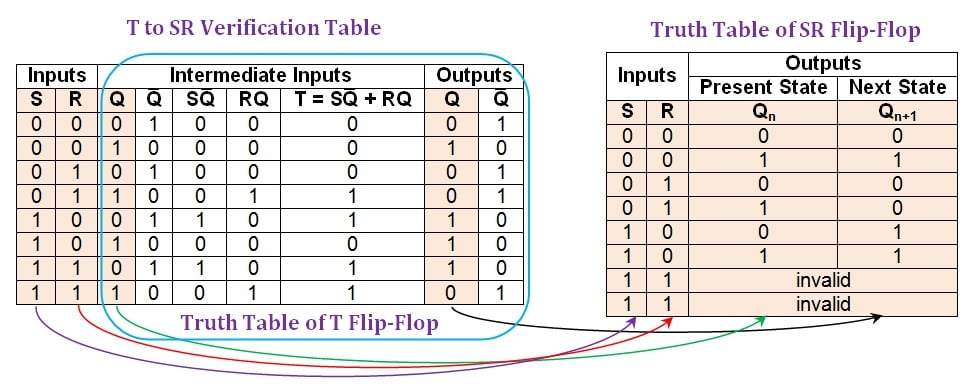##### Figure 4: Comparison between the T-to-SR verification table and the truth table of an SR flip-flop. Click to enlarge.

Figure 4 shows that the values in the first, second, third, and eighth columns (shaded in beige) of the T-to-SR verification table are consistent with those in the SR flip-flop's truth table. Thus, the conversion process was successful. The last two rows at first seem inconsistent, but they are indeed acceptable because an SR flip-flop's outputs can be either high or low when both inputs are logic high. Actually, the converted T flip-flop is better than an SR flip-flop because it has predictable output states even for the invalid input combination.

### Conversion of a T to a JK Flip-Flop

We begin with the T-to-JK conversion table (see Figure 5), which combines the information in the JK flip-flop's truth table and the T flip-flop's excitation table.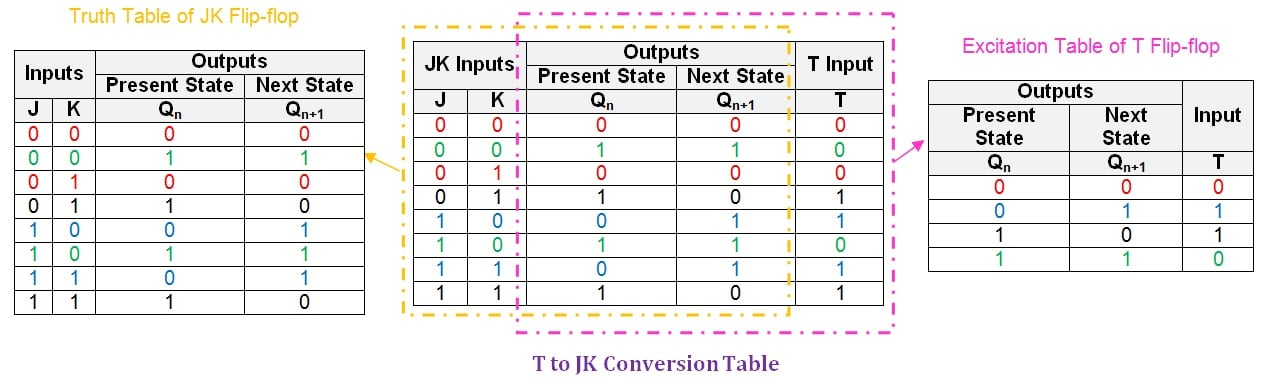##### Figure 5: T-to-JK conversion table. Click to enlarge.

Next, we need to obtain the simplified Boolean expression for the T input in terms of J, K, and Qn.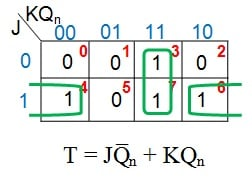##### Figure 6: K-map simplification for the T input in terms of J, K, and Qn

Figure 6 shows the expression for the T input as JQ̅n + KQn. This means that to convert the T flip-flop into a JK flip-flop, the T input is driven by the output of a two-input OR gate which has as inputs

1. J ANDed with the negation of the present-state Qn, i.e., Q̅n
2. K ANDed with the present-state, Qn

Thus, we will need two AND gates and one OR gate, as shown in Figure 7.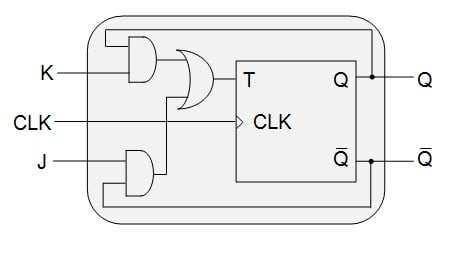##### Figure 7: T flip-flop designed to behave as a JK flip-flop

The final step is to verify whether the system behaves as we expect it to. This can be done using a T-to-JK verification table, shown in Figure 8. Here we can compare the entries in the verification table to the truth table of the JK flip-flop.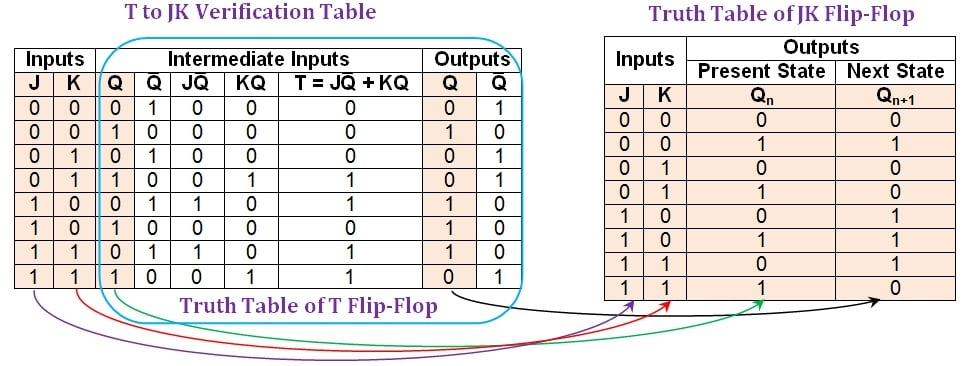##### Figure 8: Comparison between the T-to-JK verification table and the truth table of a JK flip-flop. Click to enlarge.

The entries in the first, second, third, and eighth columns (shaded in beige) of the T-to-JK verification table agree with those in the truth table of the JK flip-flop. This indicates that the given T flip-flop has become functionally equivalent to the desired JK flip-flop.

### Conversion of a T to a D Flip-Flop

We begin by writing the T-to-D conversion table (see Figure 9).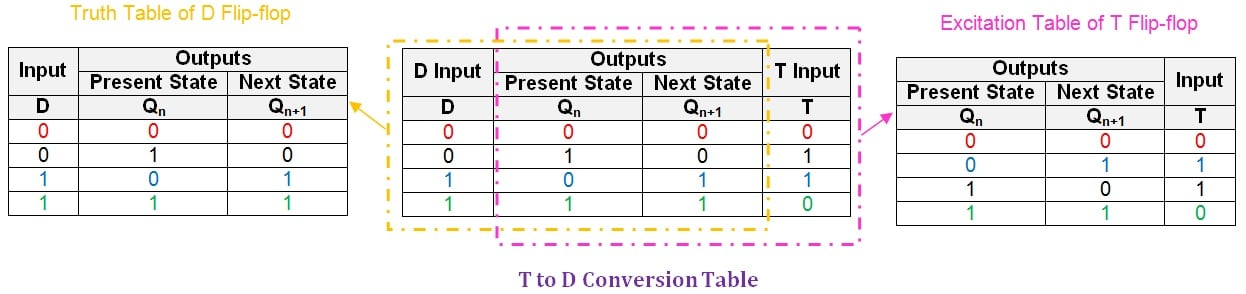##### Figure 9: T-to-D conversion table. Click to enlarge.

Once this is done, we need to express the input, T, in terms of the user-defined input, D, and the flip-flop's present-state, Qn. We will again use the K-map simplification technique.

##### Figure 10: K-map simplification for the T input in terms of D and Qn

Figure 10 shows that, in order to make the given T flip-flop functionally equivalent to a D flip-flop, we need to drive its input pin, T, with the output of an XOR gate whose inputs are D and Qn. This will lead to the new digital system shown in Figure 11(a). Figure 11(b) shows a system which is functionally equivalent to that of Figure 11(a) but is designed using only NOT, AND, and OR gates.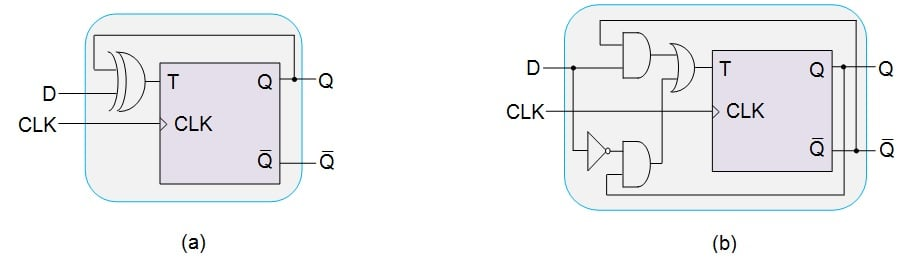##### Figure 11: T flip-flop designed to behave as a D flip-flop using (a) an XOR gate and (b) NOT, AND, and OR gates

Finally, in order to ensure that the designed system behaves as expected, we will write a T-to-D verification table, shown in Figure 12.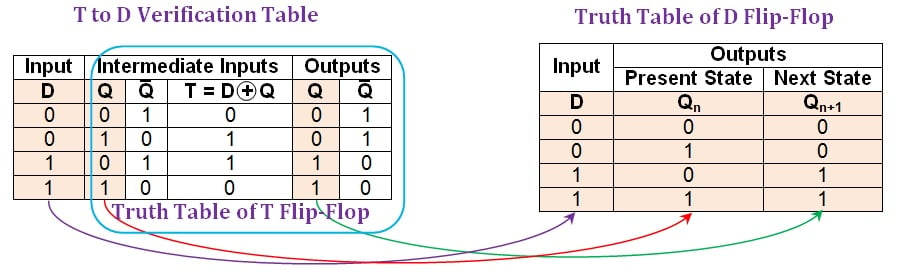##### Figure 12: Comparison between the T-to-D verification table and the truth table of a D flip-flop. Click to enlarge.

Figure 12 shows that the entries in the first, second, and fifth columns (shaded in beige) of the T-to-D verification table are the same as those in the D flip-flop's truth table. Thus, we have successfully converted the given T flip-flop into a D-type flip-flop.

Note that although we have presented the verification for the system shown in Figure 11(a), the conclusion is valid for the system in Figure 11(b) because the two systems are logically equivalent: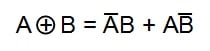### Summary

In this article, we have explained the process of converting a T flip-flop into SR-, JK-, and D-type flip-flops. We have also verified our new systems in order to confirm that the conversion processes were successful.